### Home > CALC > Chapter 12 > Lesson 12.1.3 > Problem12-38

12-38.
1. The diagram below shows a slope field. Homework Help ✎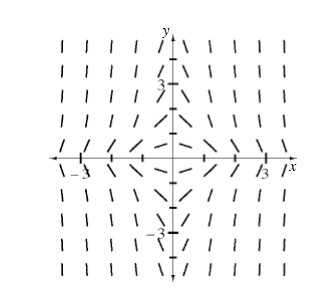1. Explain why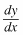must be a function of both x and y.

2. Sketch a solution curve that passes through (0, 3).

3. The slope field is for the differential equation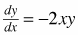. Find the equation of your solution curve.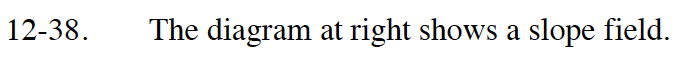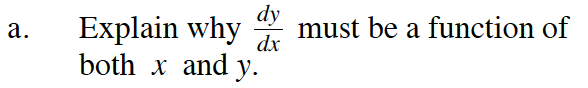Are the slopes in the diagram parallel anywhere?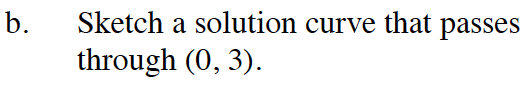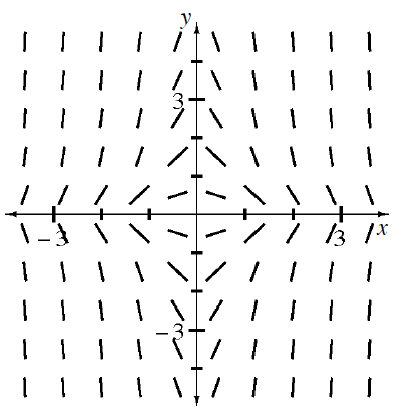Your curve should look like a bell-shaped curve with a maximum at (0, 3) and a horizontal asymptote of y = 0.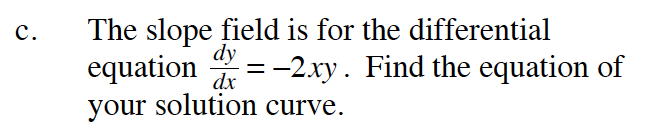Once you integrate you should have a '+ C'. Use the point (0, 3) to solve for the value of C.